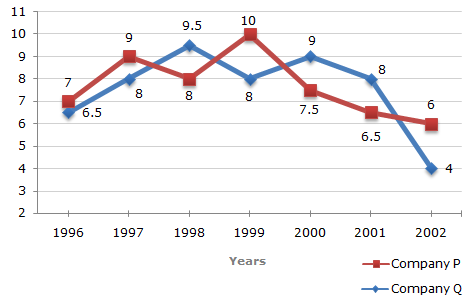# Data Interpretation - Line Charts

Exercise : Line Charts - Line Chart 8
Directions to Solve

Two different finance companies declare fixed annual rate of interest on the amounts invested with them by investors. The rate of interest offered by these companies may differ from year to year depending on the variation in the economy of the country and the banks rate of interest. The annual rate of interest offered by the two Companies P and Q over the years are shown by the line graph provided below.

Annual Rate of Interest Offered by Two Finance Companies Over the Years.1.
A sum of Rs. 4.75 lakhs was invested in Company Q in 1999 for one year. How much more interest would have been earned if the sum was invested in Company P?
Rs. 19,000
Rs. 14,250
Rs. 11,750
Rs. 9500
Explanation:

 Difference = Rs. [(10% of 4.75) - (8% of 4.75)] lakhs = Rs. (2% of 4.75) lakhs = Rs. 0.095 lakhs = Rs. 9500.

2.
If two different amounts in the ratio 8:9 are invested in Companies P and Q respectively in 2002, then the amounts received after one year as interests from Companies P and Q are respectively in the ratio?
2:3
3:4
6:7
4:3
Explanation:

Let the amounts invested in 2002 in Companies P and Q be Rs. 8x and Rs. 9x respectively.

Then, interest received after one year from Company P = Rs. (6% of 8x)
 = Rs. 48 x. 100
and interest received after one year from Company Q = Rs. (4% of 9x)
 = Rs. 36 x. 100Required ratio =48 x100
= 4 .36 x100
3

3.
In 2000, a part of Rs. 30 lakhs was invested in Company P and the rest was invested in Company Q for one year. The total interest received was Rs. 2.43 lakhs. What was the amount invested in Company P?
Rs. 9 lakhs
Rs. 11 lakhs
Rs. 12 lakhs
Rs. 18 lakhs
Explanation:

Let Rs. x lakhs be invested in Company P in 2000, the amount invested in Company Q in 2000 = Rs. (30 - x) lakhs.

Total interest received from the two Companies after 1 year

= Rs. [(7.5% of x) + {9% of (30 - x)}] lakhs

 = Rs.2.7 -1.5xlakhs. 1002.7 -1.5x= 2.43x = 18. 100

4.
An investor invested a sum of Rs. 12 lakhs in Company P in 1998. The total amount received after one year was re-invested in the same Company for one more year. The total appreciation received by the investor on his investment was?
Rs. 2,96,200
Rs. 2,42,200
Rs. 2,25,600
Rs. 2,16,000
Explanation:

Amount received from Company P after one year (i.e., in 199) on investing Rs. 12 lakhs in it

= Rs. [12 + (8% of 12)] lakhs

= Rs. 12.96 lakhs.

Amount received from Company P after one year on investing Rs. 12.96 lakhs in the year 1999

= Rs. [12.96 + (10% of 12.96)] lakhs

= Rs. 14.256.

Appreciation received on investment during the period of two years

= Rs. (14.256 - 12) lakhs

= Rs. 2.256 lakhs

= Rs. 2,25,600.

5.
An investor invested Rs. 5 lakhs in Company Q in 1996. After one year, the entire amount along with the interest was transferred as investment to Company P in 1997 for one year. What amount will be received from Company P, by the investor?
Rs. 5,94,550
Rs. 5,80,425
Rs. 5,77,800
Rs. 5,77,500
Explanation:

Amount received from Company Q after one year on investment of Rs. 5 lakhs in the year 1996

= Rs. [5 + (6.5% of 5)] lakhs

= Rs. 5.325 lakhs.

Amount received from Company P after one year on investment of Rs. 5.325 lakhs in the year 1997

= Rs. [5.325 + (9% of 5.325)] lakhs

= Rs. 5.80425 lakhs

= Rs. 5,80,425.